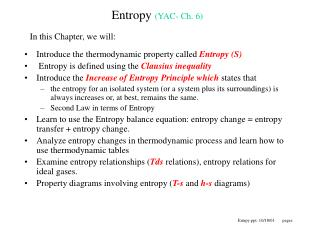DownloadDownload PresentationEntropy (YAC- Ch. 6)

# Entropy (YAC- Ch. 6)

Télécharger la présentation## Entropy (YAC- Ch. 6)

- - - - - - - - - - - - - - - - - - - - - - - - - - - E N D - - - - - - - - - - - - - - - - - - - - - - - - - - -
##### Presentation Transcript

1. Entropy (YAC- Ch. 6) In this Chapter, we will: • Introduce the thermodynamic property called Entropy (S) • Entropy is defined using the Clausius inequality • Introduce the Increase of Entropy Principle which states that • the entropy for an isolated system (or a system plus its surroundings) is always increases or, at best, remains the same. • Second Law in terms of Entropy • Learn to use the Entropy balance equation: entropy change = entropy transfer + entropy change. • Analyze entropy changes in thermodynamic process and learn how to use thermodynamic tables • Examine entropy relationships (Tds relations), entropy relations for ideal gases. • Property diagrams involving entropy (T-s and h-s diagrams) Entrpy.ppt, 10/18/01 pages

2. Entropy is a thermodynamic property; it can be viewed as a measure of disorder. i.e. More disorganized a system the higher its entropy. • Defined using Clausius inequality • where Q is the differential heat transfer & T is the absolute • temperature at the boundary where the heat transfer occurs Entropy – A Property • Clausius inequality is valid for all cycles, reversible and irreversible. • Consider a reversible Carnot cycle: • Since , i.e. it does not change if you return to the same state, • it must be a property, by defintion: • Let’s define a thermodynamic property entropy (S), such that True for a Reversible Process only

3. T any process 2 1 reversible process S Since entropy is a thermodynamic property, it has fixed values at a fixed thermodynamic states. Hence, the change, S, is determined by the initial and final state. BUT.. The change is = only for a Reversible Process Entropy (cont’d) Consider a cycle, where Process 2-1 is reversible and 1-2 may or may not be reversible

4. Increase of Entropy Principle (YAC- Ch. 6-3) Increase of Entropy Principle Entropy change Entropy Transfer (due to heat transfer) Entropy Generation The principle states that for an isolated Or a closed adiabatic Or System + Surroundings A process can only take place such that Sgen 0 where Sge = 0 for a reversible process only And Sge can never be les than zero. • Implications: • Entropy, unlike energy, is non-conservative since it is always increasing. • The entropy of the universe is continuously increasing, in other words, it is becoming disorganized and is approaching chaotic. • The entropy generation is due to the presence of irreversibilities. Therefore, the higher the entropy generation the higher the irreversibilities and, accordingly, the lower the efficiency of a device since a reversible system is the most efficient system. • The above is another statement of the second law

5. Increase of Entropy Principle is another way of stating the Second Law of Thermodynamics: • Second Law : Entropy can be created but NOT destroyed • (In contrast, the first law states: Energy is always conserved) • Note that this does not mean that the entropy of a system cannot be reduced, it can. • However, total entropy of a system + surroundings cannot be reduced • Entropy Balance is used to determine the Change in entropy of a system as follows: • Entropy change = Entropy Transfer + Entropy Generation where, • Entropy change = S = S2 - S1 • Entropy Transfer = Transfer due to Heat (Q/T) + Entropy flow due to mass flow (misi – mese) • Entropy Generation = Sgen 0 • For a Closed System: S2 - S1 = Qk /Tk + Sgen • In Rate Form:dS/dt = Qk /Tk + Sgen • For an Open System (Control Volume): • Similar to energy and mass conservation, the entropy balance equations can be simplified • Under appropriate conditions, e.g. steady state, adiabatic…. Second Law & Entropy Balance (YAC- Ch. 6-4)

6. Source 800 K Q=2000 kJ Sink 500 K Entropy Generation Example Show that heat can not be transferred from the low-temperature sink to the high-temperature source based on the increase of entropy principle. • DS(source) = 2000/800 = 2.5 (kJ/K) • DS(sink) = -2000/500 = -4 (kJ/K) • Sgen= DS(source)+ DS(sink) = -1.5(kJ/K) < 0 • It is impossible based on the entropy increase principle • Sgen0, therefore, the heat can not transfer from low-temp. to high-temp. without external work input • If the process is reversed, 2000 kJ of heat is transferred from the source to the sink, Sgen=1.5 (kJ/K) > 0, and the process can occur according to the second law • If the sink temperature is increased to 700 K, how about the entropy generation? DS(source) = -2000/800 = -2.5(kJ/K) • DS(sink) = 2000/700 = 2.86 (kJ/K) • Sgen= DS(source)+ DS(sink) = 0.36 (kJ/K) < 1.5 (kJ/K) • Entropy generation is less than when the sink temperature is 500 K, less irreversibility. Heat transfer between objects having large temperature difference generates higher degree of irreversibilities